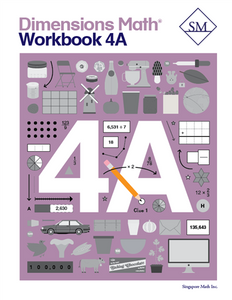# Dimensions Math Workbook 4A

• \$12.00
Unit price per

Workbooks offer the independent practice necessary for students to hone their skills. Corresponding to textbook material, lessons begin with fundamentals and progresses to more challenging problems.

Features & Components:
• Basics: Provide a review of basic concepts covered in textbooks.
• Practice: Reinforces and consolidates learning through practice problems.
• Challenge: Offers advanced enrichment through challenging problems.
• Check: Gives students a chance to confirm their understanding.
Note: Two workbooks (A and B) for each grade correspond to the two halves of the school year. Answer key not included. Soft cover. Grades PK-2 Workbooks are perforated.

Dimensions Math® Workbook 4A

Chapter 1: Numbers to One Million

Chapter 3: Multiples and Factors

Chapter 4: Multiplication

Chapter 5: Division

Chapter 6: Fractions

Chapter 7: Adding and Subtracting Fractions

Chapter 8: Multiplying a Fraction and a Whole Number

Chapter 9: Line Graphs and Line Plots